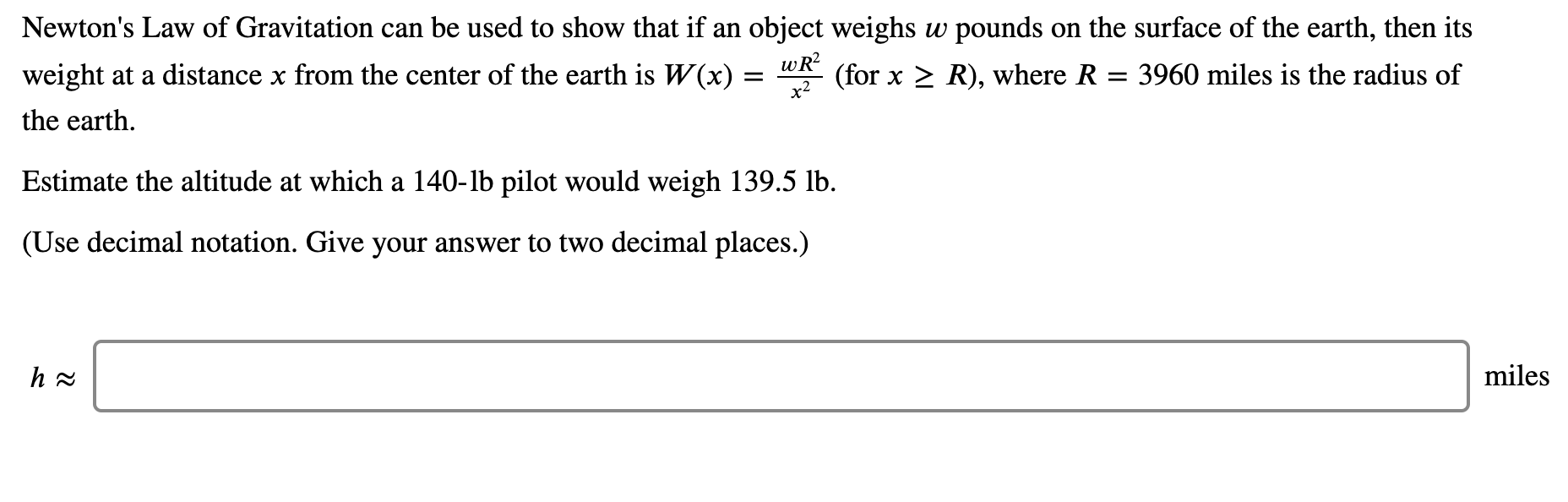# Newton's Law of Gravitation can be used to show that if an object weighs w pounds on the surface of the earth, then its weight at a distance x from the center of the earth is W(x) = wR? (for x > R), where R = 3960 miles is the radius of the earth. Estimate the altitude at which a 140-lb pilot would weigh 139.5 lb. (Use decimal notation. Give your answer to two decimal places.)

Questionhelp_outlineImage TranscriptioncloseNewton's Law of Gravitation can be used to show that if an object weighs w pounds on the surface of the earth, then its weight at a distance x from the center of the earth is W(x) = wR? (for x > R), where R = 3960 miles is the radius of the earth. Estimate the altitude at which a 140-lb pilot would weigh 139.5 lb. (Use decimal notation. Give your answer to two decimal places.) fullscreen

### Want to see this answer and more?

Experts are waiting 24/7 to provide step-by-step solutions in as fast as 30 minutes!*

*Response times may vary by subject and question complexity. Median response time is 34 minutes for paid subscribers and may be longer for promotional offers.
Tagged in
Science
Physics

### Gravitation